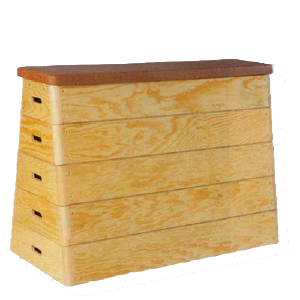## Use BASE to not ruin what you already haveWe used to use the `APPEND` and `INSERT` commands to add rows to internal tables. Now that ABAP has grown, we can use `VALUE`, `REDUCE`, `CORRESPONDING`, etc. But what if the internal table already has rows and we don’t want to lose them?

In that case you use `BASE`.

``````output_lines = CORRESPONDING #( input_lines ).
output_lines = CORRESPONDING #( BASE ( output_lines ) input_lines ).
``````

The difference between the first and second lines is that, while in the first the content of `output_lines` is crushed in the operation, in the second it is preserved and the new lines resulting from the `CORRESPONDING` operation are added after the existing ones.

Luís Rocha suggested this topic and sent me the following examples:

``````LOOP AT lines ASSIGNING FIELD-SYMBOL(<line>).
result = VALUE #(
BASE result
sign = 'I' option = 'EQ' high = ''
( low = <line>-value ) ).
ENDLOOP.
``````

In the example above you are building a range iteratively. Note that in this particular case you could alternatively have added `FOR EACH` to `VALUE`. But then you wouldn’t learn how to use `BASE`, would you?

In the example below, you add a few more lines of HTML to a table that already has some:

``````it_html = VALUE #(
( |<html>| )
( |    <meta http-equiv="content-type">| )
( |    <script type="text/javascript">| )
( |      function onKeyDown(f)| )
( |      \{ if(event.keyCode == 13)| )
( |         \{ document[f.name].submit();  | )
( |         \}| )
( |      \}| )
( |    </script>| )
( |    <style type="text/css">| )
).

" (...)

it_html = VALUE #( BASE it_html
( |      </style> | )